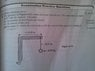# Rotating pendulum hang from a wooden bar with a revolution speed of 0.15 rev/s.

• JLABBER
In summary, the conversation discusses a problem with a pendulum attached to a wooden bar and a string on a table. The goal is to find the angle the pendulum makes with respect to the vertical axis given the revolution speed. The conversation covers different attempts at solving the problem and suggests using numerical methods such as iteration to find a valid answer. It also mentions other methods such as graphing to solve implicit equations.

## Homework Statement

Part c: A pendulum is attached to a 0.15m wooden bar sticked horizontally to a table by a string of 0.12m. If the system is revolved with a revolution speed of 1.5 rev per second, what is the angle θ the pendulum make with respect to the vertical axis?

## Homework Equations

Tsin θ =mrw^2
Tcos θ = mg
where r=0.15 +0.12 sin θ

## The Attempt at a Solution

To identify my mistake at how to solve this question as I can't get the answer provided which is 85.7 degree using my original equation. Instead, i get a value of 209 degree which sounded so impossible to occur.

Thanks for help.

#### Attachments

•379978_1998682420653_1651291436_31579803_1126692534_n.jpg
34 KB · Views: 376
You are right, the solution you got is not possible. Show your derivation. The question can not be answered exactly, you need some numerical method. Check the validity of the solution given. I think it is also wrong.

ehild

Okay, i will check. Thanks a lot. I think the question is taken directly from a book by my lecturer. And the answer too. What do you mean by numerical method? Do you mean that my solution is right but not possible? And this question can be solved with a valid possible answer through that numerical method?

For my way of solving,
First i divide the first equation by the second equation and i get
tan theta=rw^2/g
Then, i substitute r= 0.15 + 0.12 sin theta into the new equation,
tan theta = (0.15 + 0.12 sin theta) (1.5 X 2pi) ,
After that, i try to rearrange and solve for the theta, but i have no way to get the answer because there is sin and tan simultaneously, i have no point of solving it. But, using a calculator, I key in the equations, and get an ans of -871 degree of the equation. Is my equation correct anyway?

JLABBER said:
For my way of solving,
First i divide the first equation by the second equation and i get
tan theta=rw^2/g

That is correct.

JLABBER said:
Then, i substitute r= 0.15 + 0.12 sin theta into the new equation,
tan theta = (0.15 + 0.12 sin theta) (1.5 X 2pi)2/g
You missed a square and dividing by g.

JLABBER said:
After that, i try to rearrange and solve for the theta, but i have no way to get the answer because there is sin and tan simultaneously, i have no point of solving it. But, using a calculator, I key in the equations, and get an ans of -871 degree of the equation. Is my equation correct anyway?

-871 degrees? ... That is almost three rounds clockwise:rofl:

Correct the last equation and substitute the given angle, than try 67 degrees.

ehild

Wow, exactly 67 degree can solve for the equation. But, how should i solve equation involving sin and tan simultaneously? I do not have a single idea of solving it. Should we first changing tan into sin/cos?

You can do it by iteration. Write the equation in form of

tan(θ ) = A(1+Bsin(θ ))

It will be

tan(θ ) = 1.3596(1+0.8sin(θ ))

for the given data.
Start with θ=0, and evaluate the right-hand side. You get tan(θ), find inverse tangent. This will be your θ for the next step. find the sine, evaluate the RHS , get arctan, and repeat till the result does not change within 3 digits. Try, it is funehild

Okay, i will try. Such a new knowledge for me, thanks a lot.

You are welcome. The method of iteration is very useful, although it does not work always.

ehild

I see. Ya,One more question, for this method, does it apply only to equation when tan theta= A(1+sin theta)?

It applies for other cases in form x=f(x), but the magnitude of the derivative of f has to be less than 1 (|f'|<1).
There are other methods to solve implicit equations numerically. It is always very useful to plot both sides and find the crosspoint graphically.

ehild